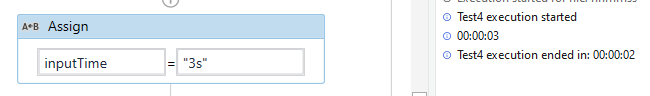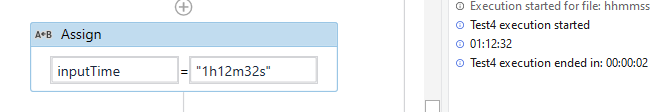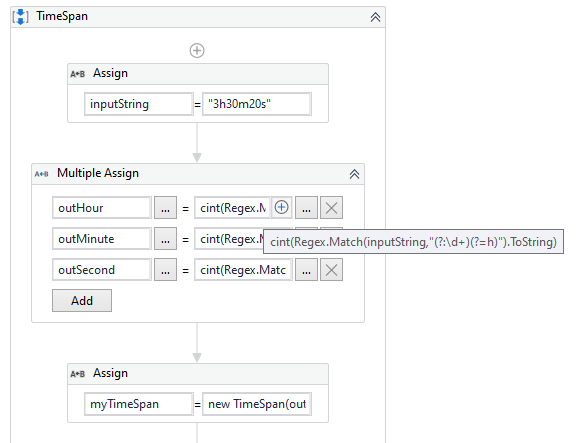# How to Convert Time In a Specific Format

Hello good people,

Is there any way to convert a time value for example, 3s to hh:mm:ss format?

More examples,

3s = 00:00:03
4m2s = 00:04:02
1h12m32s = 01:12:32

@AKM_Robinuzzaman

What is the format of the input?
is it a string?

Yes, its a string.

You can create TimeSpan for the desired output type, by parsing your input values into variables.

Let me know if that can work for you.

Here my input is in string format. Can you please give me an example of how did you get desired output using timeSpan?

Thanks!

@AKM_Robinuzzaman

You can proceed like this``````TimeSpan.FromSeconds(
(CInt("0"+System.Text.RegularExpressions.Regex.Match(inputTime, "\d{1,2}(?=h)").ToString)*60*60)+
(CInt("0"+System.Text.RegularExpressions.Regex.Match(inputTime, "\d{1,2}(?=m)").ToString)*60)+
CInt(System.Text.RegularExpressions.Regex.Match(inputTime, "\d{1,2}(?=s)").ToString)
)
``````

# Output2 Likes

Here, I used regex to parse the string into hour, minute and second variables. You can use “if” for the values that doesn’t exist and assign them to zero.
Edit: example for if
`If(not String.IsNullOrEmpty(Regex.Match(inputString,"(?:\d+)(?=h)").ToString),cint(Regex.Match(inputString,"(?:\d+)(?=h)").ToString),0)`

``````cint(Regex.Match(inputString,"(?:\d+)(?=h)").ToString)
new TimeSpan(outHour, outMinute, outSecond)
``````1 Like

This is working perfectly for me. Thank you so much for your time. Really appreciate it.

Regards,
Robin

@sarikayaebru , Thank you so much for you time. Really appreciate the effort.

Regards,
Robin

1 Like

This topic was automatically closed 3 days after the last reply. New replies are no longer allowed.